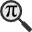## General

Display information for equation id:math.219374.34 on revision:219374

* Page found: Euler's formula (eq math.219374.34)

(force rerendering)

Cannot find the equation data in the database. Fetching from revision text.

Occurrences on the following pages:

Hash: a6303e2ca49f70fa9e65808a0d2d5626

TeX (original user input):

e^z


TeX (checked):

e^{z}


### LaTeXML (experimental; uses MathML) rendering

MathML (845 B / 338 B) :$e^{z}$
<math xmlns="http://www.w3.org/1998/Math/MathML" id="p1.1.m1.1" class="ltx_Math" alttext="{\displaystyle e^{z}}" display="inline">
<semantics id="p1.1.m1.1a">
<msup id="p1.1.m1.1.3" xref="p1.1.m1.1.3.cmml">
<mi id="p1.1.m1.1.1" xref="p1.1.m1.1.1.cmml">e</mi>
<mi id="p1.1.m1.1.2.1" xref="p1.1.m1.1.2.1.cmml">z</mi>
</msup>
<annotation-xml encoding="MathML-Content" id="p1.1.m1.1b">
<apply id="p1.1.m1.1.3.cmml" xref="p1.1.m1.1.3">
<csymbol cd="ambiguous" id="p1.1.m1.1.3.1.cmml" xref="p1.1.m1.1.3">superscript</csymbol>
<ci id="p1.1.m1.1.1.cmml" xref="p1.1.m1.1.1">𝑒</ci>
<ci id="p1.1.m1.1.2.1.cmml" xref="p1.1.m1.1.2.1">𝑧</ci>
</apply>
</annotation-xml>
<annotation encoding="application/x-tex" id="p1.1.m1.1c">{\displaystyle e^{z}}</annotation>
</semantics>
[/itex]


SVG (1.854 KB / 982 B) :

### MathML with SVG or PNG fallback (recommended for modern browsers and accessibility tools) rendering

SVG image empty. Force Re-Rendering

SVG (0 B / 8 B) :

PNG (0 B / 8 B) :

$e^{z}$## Translations to Computer Algebra Systems

### Translation to Maple

In Maple: (e)^(z)

e: the mathematical constant e == Napier's constant == 2.71828182845... was translated to: e

exp(1): You use a typical letter for a constant [the mathematical constant e == Napier's constant == 2.71828182845...].

We keep it like it is! But you should know that Maple uses exp(1) for this constant.

If you want to translate it as a constant, use the corresponding DLMF macro \expe

### Translation to Mathematica

In Mathematica: (e)^(z)

E: You use a typical letter for a constant [the mathematical constant e == Napier's constant == 2.71828182845...].

We keep it like it is! But you should know that Mathematica uses E for this constant.

If you want to translate it as a constant, use the corresponding DLMF macro \expe

e: the mathematical constant e == Napier's constant == 2.71828182845... was translated to: e

## Similar pages

Calculated based on the variables occurring on the entire Euler's formula page

## Identifiers

• $e$• $z$### MathML observations

0results

0results

no statistics present please run the maintenance script ExtractFeatures.php

0 results

0 results